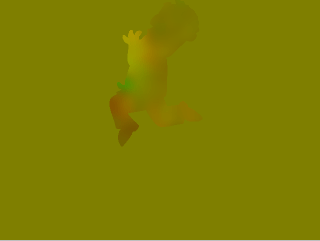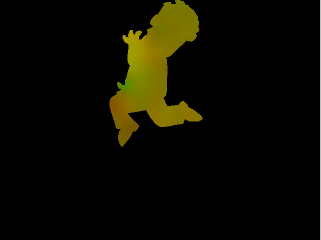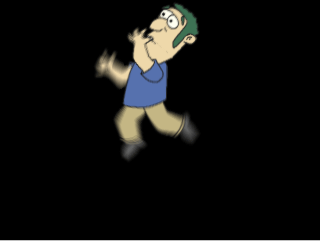# How is alpha used in your motion vectors?

RSMB Vectors assumes that the vector information at a particular pixel is not valid if the the alpha channel in the motion vector image is less than full-on at a particular pixel. Note that transparent source imagery (not motion vector imagery) will be blurred properly, because the alpha for the color source is independent of the alpha for the motion vector image sequence.

It is important to set the alpha to full-on values for the motion vector images where motion vectors are known to be valid. Areas of the motion vector image where the motion vectors are not known (or are known to be bad) should have alpha set to zero or any value other than full-on. It is imporant to categorize each pixel in the motion vector file as good or bad for RSMB Vectors to work properly. For example, when rendering vectors from a 3D animation system it is useful to set the alpha channel to the same alpha channel that is used for compositing the element.

For example, lets say we have the following image created from an animation system.

Let’s say we have the following image created from an animation system.Examples of incorrect and correct setup of motion vector filesIncorrect Motion Vector creation: Areas of unknown motion vector data set to (0,0) displacement.Correct Motion Vector creation: Alpha of areas of unknown vector data set to 0.Animation blurred with motion vector image above in ReelSmart Motion Blur. Areas with (0,0) displacement do not get blurred. As such, notice the harsh edges around the hand and right foot.Animation blurred with the vector file. Areas where the vector information not known are now blurred appropriately.